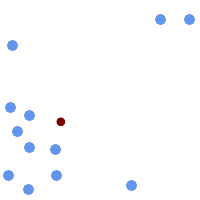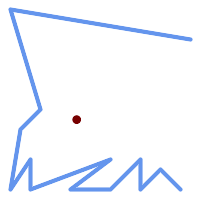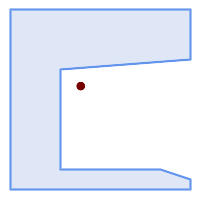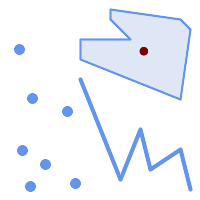## Name

ST_Centroid — Returns the geometric center of a geometry.

## Synopsis

`geometry ST_Centroid(`geometry g1`)`;

`geography ST_Centroid(`geography g1, boolean use_spheroid = true`)`;

## Description

Computes a point which is the geometric center of mass of a geometry. For [`MULTI`]`POINT`s, the centroid is the arithmetic mean of the input coordinates. For [`MULTI`]`LINESTRING`s, the centroid is computed using the weighted length of each line segment. For [`MULTI`]`POLYGON`s, the centroid is computed in terms of area. If an empty geometry is supplied, an empty `GEOMETRYCOLLECTION` is returned. If `NULL` is supplied, `NULL` is returned. If `CIRCULARSTRING` or `COMPOUNDCURVE` are supplied, they are converted to linestring with CurveToLine first, then same than for `LINESTRING`

For mixed-dimension input, the result is equal to the centroid of the component Geometries of highest dimension (since the lower-dimension geometries contribute zero "weight" to the centroid).

Note that for polygonal geometries the centroid does not necessarily lie in the interior of the polygon. For example, see the diagram below of the centroid of a C-shaped polygon. To construct a point guaranteed to lie in the interior of a polygon use ST_PointOnSurface.

New in 2.3.0 : supports `CIRCULARSTRING` and `COMPOUNDCURVE` (using CurveToLine)

Availability: 2.4.0 support for geography was introduced.This method implements the OGC Simple Features Implementation Specification for SQL 1.1.This method implements the SQL/MM specification.

SQL-MM 3: 8.1.4, 9.5.5

## Examples

In the following illustrations the red dot is the centroid of the source geometry.Centroid of a `MULTIPOINT`Centroid of a `LINESTRING`Centroid of a `POLYGON`Centroid of a `GEOMETRYCOLLECTION`
```SELECT ST_AsText(ST_Centroid('MULTIPOINT ( -1 0, -1 2, -1 3, -1 4, -1 7, 0 1, 0 3, 1 1, 2 0, 6 0, 7 8, 9 8, 10 6 )'));
st_astext
------------------------------------------
POINT(2.30769230769231 3.30769230769231)
(1 row)

SELECT ST_AsText(ST_centroid(g))
FROM  ST_GeomFromText('CIRCULARSTRING(0 2, -1 1,0 0, 0.5 0, 1 0, 2 1, 1 2, 0.5 2, 0 2)')  AS g ;
------------------------------------------
POINT(0.5 1)

SELECT ST_AsText(ST_centroid(g))
FROM  ST_GeomFromText('COMPOUNDCURVE(CIRCULARSTRING(0 2, -1 1,0 0),(0 0, 0.5 0, 1 0),CIRCULARSTRING( 1 0, 2 1, 1 2),(1 2, 0.5 2, 0 2))' ) AS g;
------------------------------------------
POINT(0.5 1)

```